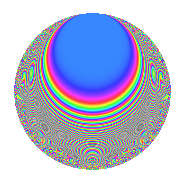# Properties

 Label 109.2.fLevel 109 Weight 2 Character orbit f Rep. character $$\chi_{109}(16,\cdot)$$ Character field $$\Q(\zeta_{9})$$ Dimension 42 Newform subspaces 1 Sturm bound 18 Trace bound 0

# Related objects

## Defining parameters

 Level: $$N$$ = $$109$$ Weight: $$k$$ = $$2$$ Character orbit: $$[\chi]$$ = 109.f (of order $$9$$ and degree $$6$$) Character conductor: $$\operatorname{cond}(\chi)$$ = $$109$$ Character field: $$\Q(\zeta_{9})$$ Newform subspaces: $$1$$ Sturm bound: $$18$$ Trace bound: $$0$$

## Dimensions

The following table gives the dimensions of various subspaces of $$M_{2}(109, [\chi])$$.

Total New Old
Modular forms 54 54 0
Cusp forms 42 42 0
Eisenstein series 12 12 0

## Trace form

 $$42q - 6q^{3} - 12q^{4} - 6q^{5} + 12q^{6} + 3q^{7} - 12q^{8} - 12q^{9} + O(q^{10})$$ $$42q - 6q^{3} - 12q^{4} - 6q^{5} + 12q^{6} + 3q^{7} - 12q^{8} - 12q^{9} + 15q^{10} - 15q^{11} + 9q^{12} - 30q^{13} + 3q^{14} + 6q^{16} - 3q^{17} - 27q^{18} - 3q^{19} - 30q^{20} - 3q^{21} - 18q^{22} + 6q^{23} - 12q^{24} + 6q^{25} + 15q^{26} + 3q^{27} + 66q^{28} + 3q^{30} + 6q^{31} + 12q^{32} + 24q^{33} - 21q^{34} - 54q^{35} + 21q^{36} - 24q^{37} + 27q^{38} + 18q^{39} - 24q^{40} - 30q^{41} + 12q^{42} + 9q^{43} + 36q^{44} + 12q^{45} - 12q^{46} - 42q^{47} - 27q^{48} + 15q^{49} + 3q^{50} - 12q^{51} - 3q^{52} + 3q^{53} - 36q^{54} + 21q^{55} + 57q^{56} - 15q^{57} - 24q^{58} + 18q^{59} + 33q^{60} + 6q^{61} + 78q^{62} - 48q^{63} - 12q^{64} + 3q^{65} - 15q^{66} - 6q^{67} + 66q^{68} + 15q^{69} + 39q^{70} + 15q^{71} - 9q^{72} + 66q^{73} - 24q^{74} + 24q^{75} - 96q^{76} - 39q^{77} - 3q^{78} + 18q^{79} - 3q^{80} - 15q^{81} + 21q^{82} + 21q^{83} + 87q^{84} + 120q^{85} - 15q^{86} + 12q^{87} - 48q^{88} + 15q^{89} + 24q^{90} + 63q^{92} - 75q^{93} - 30q^{94} + 15q^{95} - 21q^{96} + 48q^{97} - 126q^{98} + 39q^{99} + O(q^{100})$$

## Decomposition of $$S_{2}^{\mathrm{new}}(109, [\chi])$$ into newform subspaces

Label Dim. $$A$$ Field CM Traces $q$-expansion
$$a_2$$ $$a_3$$ $$a_5$$ $$a_7$$
109.2.f.a $$42$$ $$0.870$$ None $$0$$ $$-6$$ $$-6$$ $$3$$